# Cell Division Interphase Mitosis 1 Mitosis 2 Meiosis

• Slides: 28Cell Division!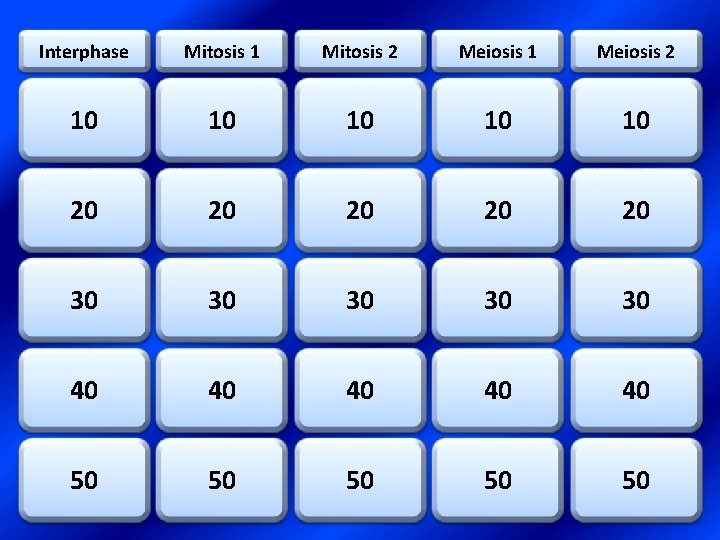Interphase Mitosis 1 Mitosis 2 Meiosis 1 Meiosis 2 10 10 10 20 20 20 30 30 30 40 40 40 50 50 50Topic 1 – 10 Points QUESTION: • What are three stages within Interphase? ANSWER: • Growth 1 (G 1), Synthesis (S), Growth 2, (G 2)Topic 1 – 20 Points QUESTION: What major event happens in the S phase? • ANSWER: • DNA replication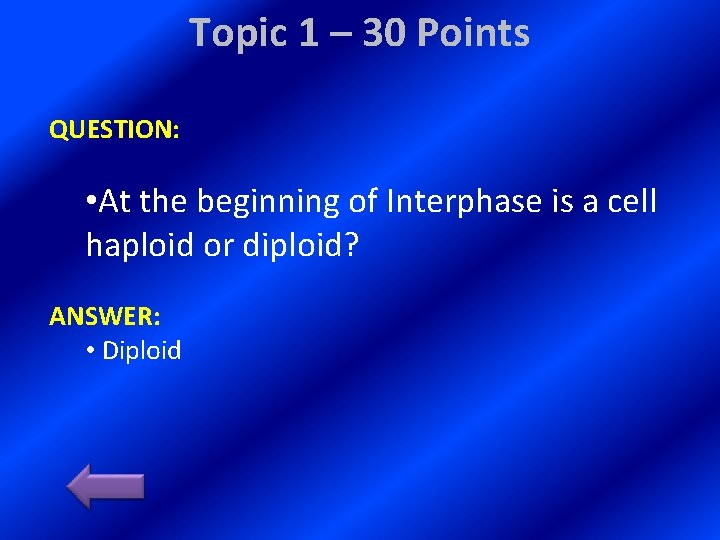Topic 1 – 30 Points QUESTION: • At the beginning of Interphase is a cell haploid or diploid? ANSWER: • DiploidTopic 1 – 40 Points QUESTION: • What allows cancer cells to divide so quickly? ANSWER: • Checkpoints are removed or ignored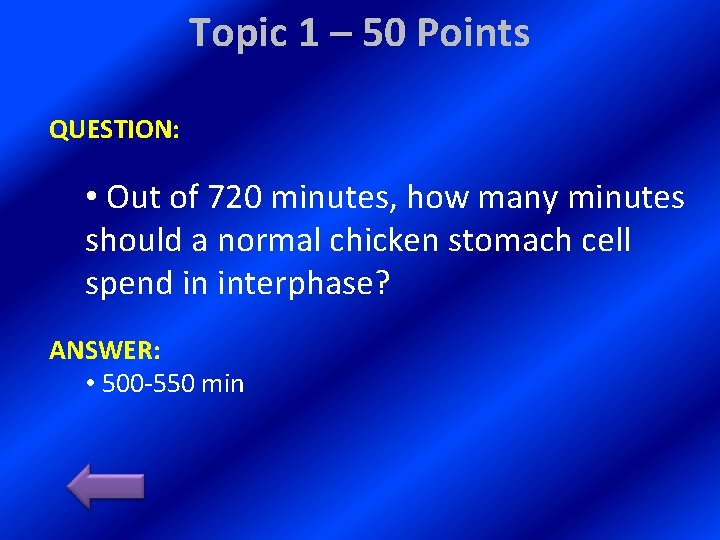Topic 1 – 50 Points QUESTION: • Out of 720 minutes, how many minutes should a normal chicken stomach cell spend in interphase? ANSWER: • 500 -550 minTopic 2 – 10 Points QUESTION: • What order? are the four phases of mitosis in ANSWER: • Prophase, Metaphase, Anaphase, Telophase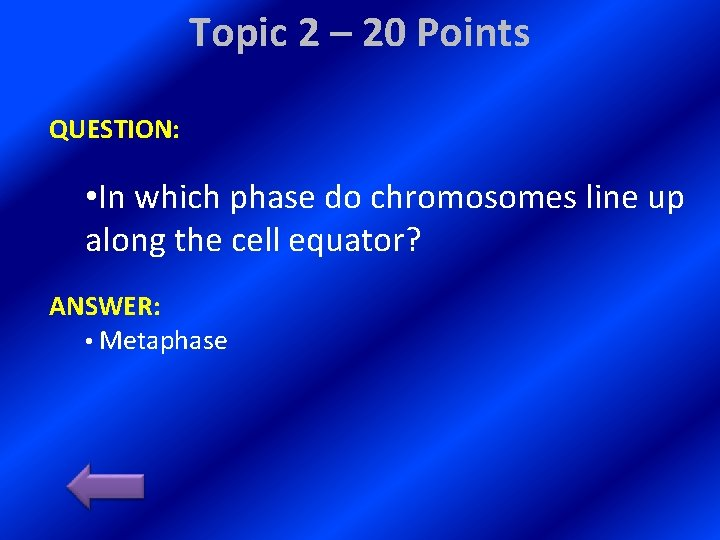Topic 2 – 20 Points QUESTION: • In which phase do chromosomes line up along the cell equator? ANSWER: • Metaphase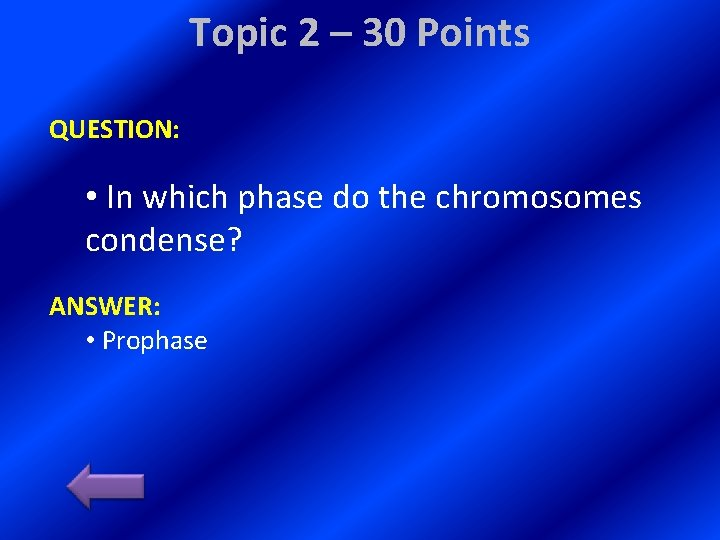Topic 2 – 30 Points QUESTION: • In which phase do the chromosomes condense? ANSWER: • Prophase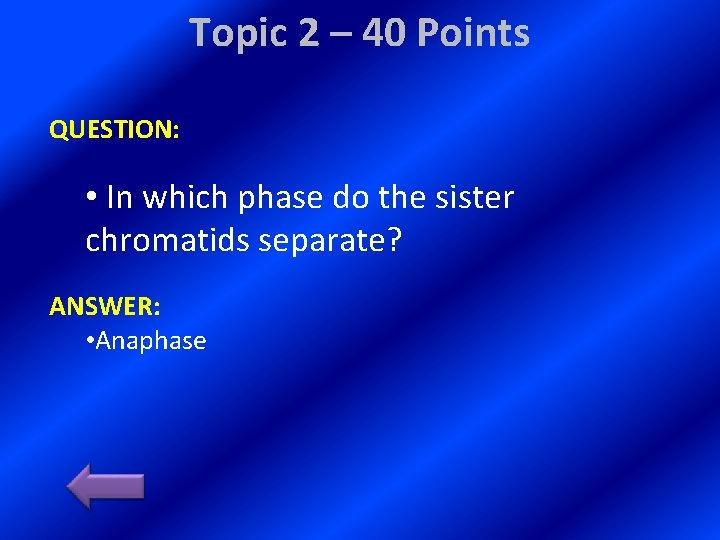Topic 2 – 40 Points QUESTION: • In which phase do the sister chromatids separate? ANSWER: • AnaphaseTopic 2 – 50 Points QUESTION: Daily Double • List three reasons for cell division. ANSWER: • Reproduction, Growth and development, tissue renewal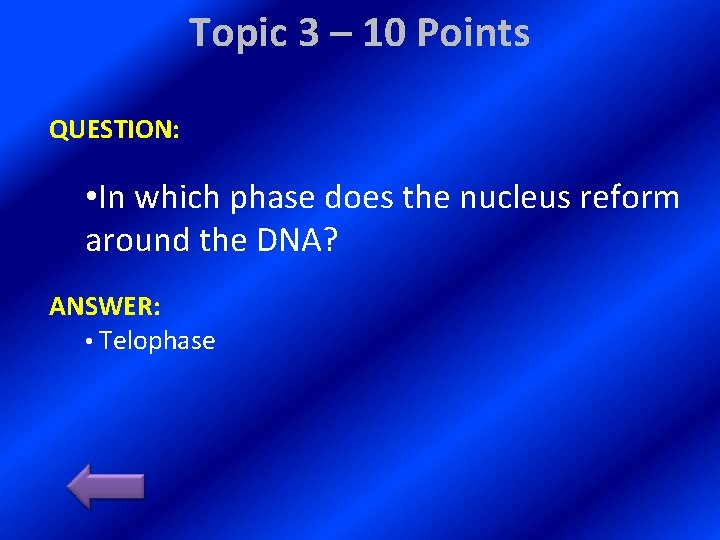Topic 3 – 10 Points QUESTION: • In which phase does the nucleus reform around the DNA? ANSWER: • TelophaseTopic 3 – 20 Points QUESTION: • In which phase do the centrioles moves to the poles? ANSWER: • Prophase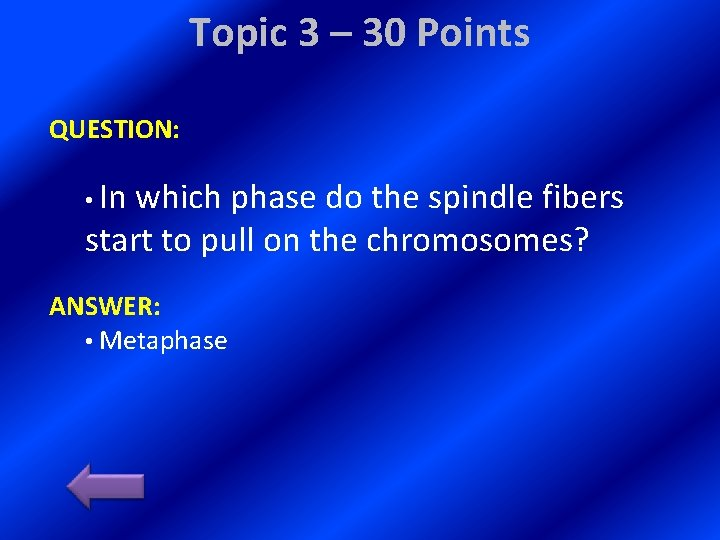Topic 3 – 30 Points QUESTION: • In which phase do the spindle fibers start to pull on the chromosomes? ANSWER: • MetaphaseTopic 3 – 40 Points QUESTION: • In which phase do the centromeres split? ANSWER: • Anaphase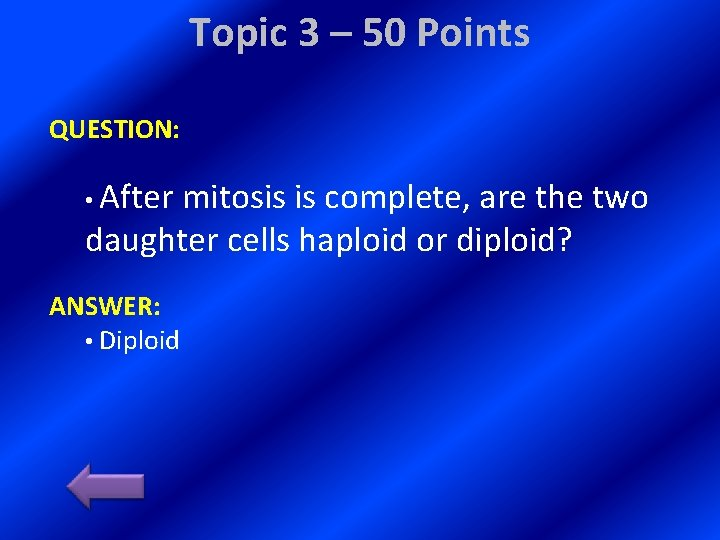Topic 3 – 50 Points QUESTION: • After mitosis is complete, are the two daughter cells haploid or diploid? ANSWER: • DiploidTopic 4 – 10 Points QUESTION: • What is it called when homologous chromosomes pair up? ANSWER: • Synapsis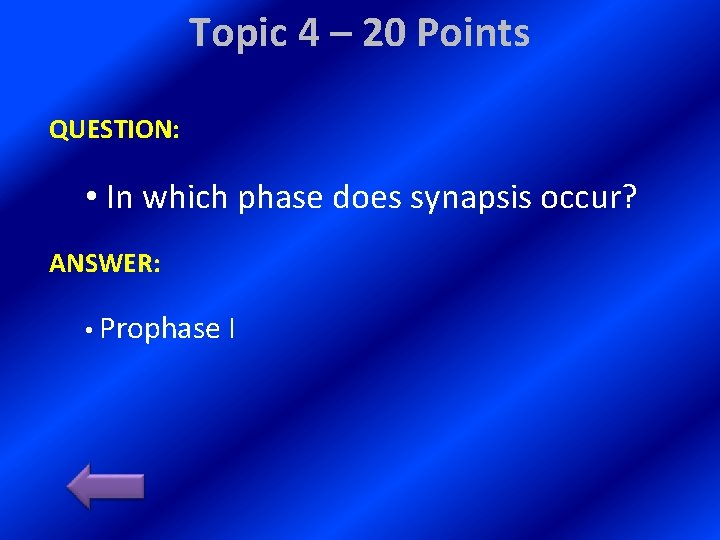Topic 4 – 20 Points QUESTION: • In which phase does synapsis occur? ANSWER: • Prophase ITopic 4 – 30 Points QUESTION: • In which phase does independent assortment occur? ANSWER: • Metaphase I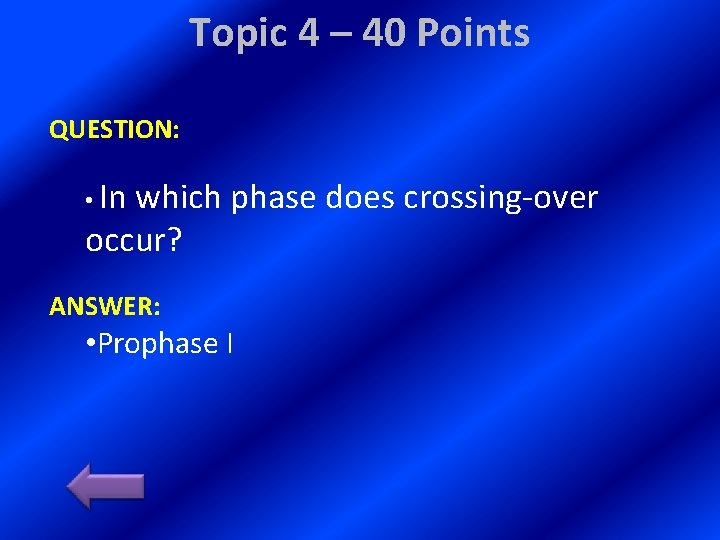Topic 4 – 40 Points QUESTION: • In which phase does crossing-over occur? ANSWER: • Prophase ITopic 4 – 50 Points QUESTION: • What are three factors that ensure genetic variability? ANSWER: • Crossing-over, independent assortment, random fertilizationTopic 5 – 10 Points QUESTION: • In which phase do the homologous chromosomes separate? ANSWER: • Anaphase I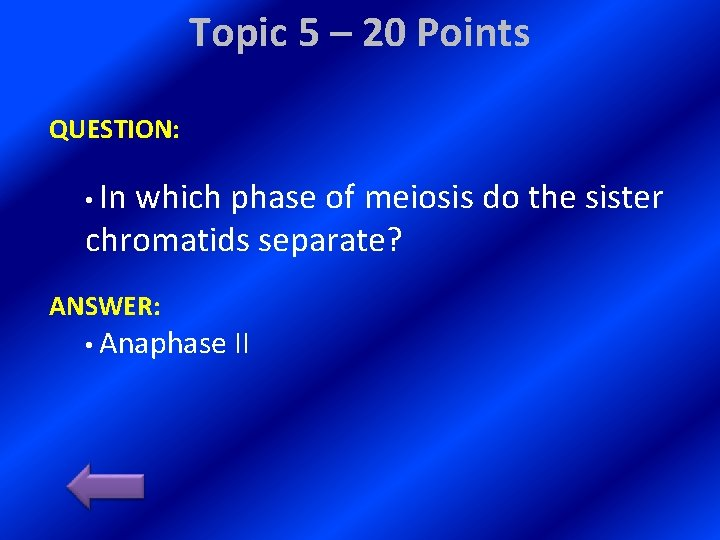Topic 5 – 20 Points QUESTION: • In which phase of meiosis do the sister chromatids separate? ANSWER: • Anaphase II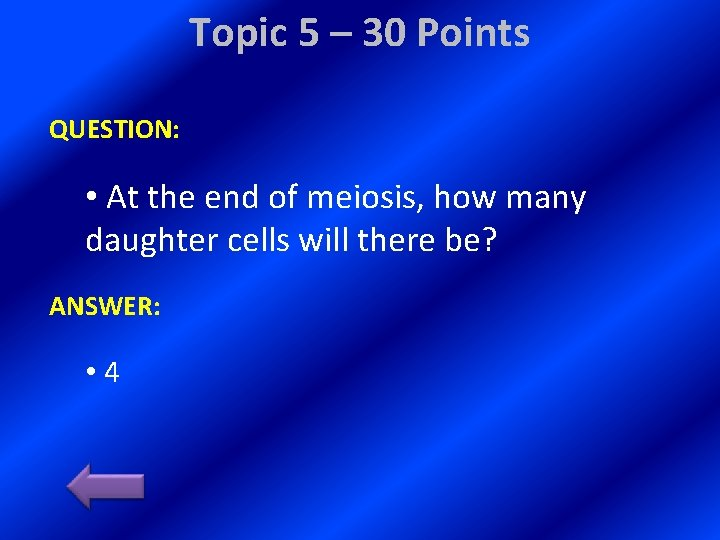Topic 5 – 30 Points QUESTION: • At the end of meiosis, how many daughter cells will there be? ANSWER: • 4Topic 5 – 40 Points QUESTION: • At the end of meiosis I, are the daughter cells haploid or diploid? ANSWER: • Haploid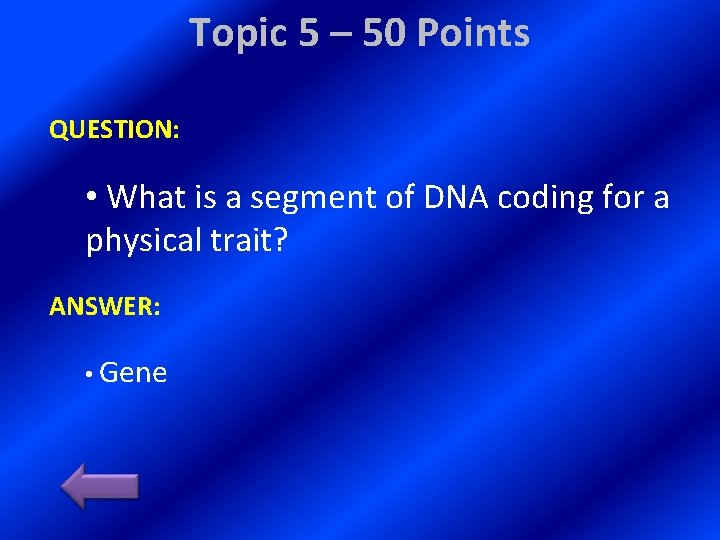Topic 5 – 50 Points QUESTION: • What is a segment of DNA coding for a physical trait? ANSWER: • Gene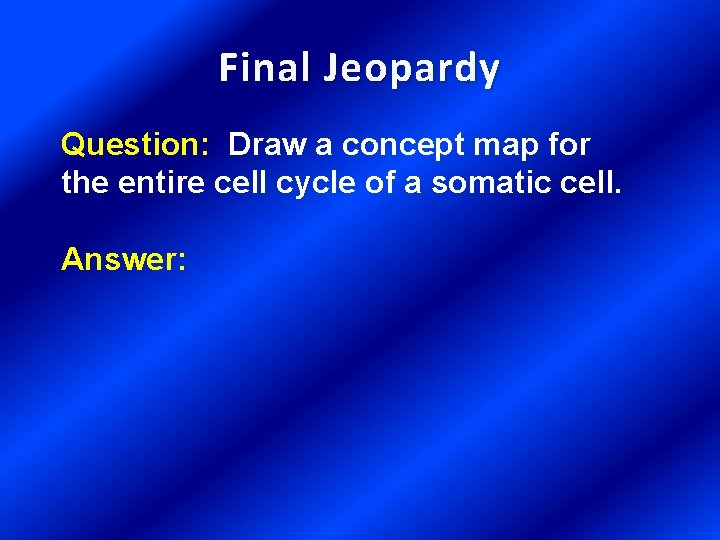Final Jeopardy Question: Draw a concept map for the entire cell cycle of a somatic cell. Answer: